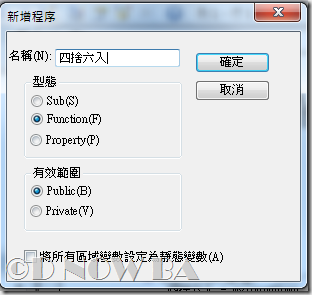## 2012年12月8日 星期六

### Excel VBA 四捨六入五成雙的設計

Excel VBA d005d1四捨六入

1.如果數字是4以下則捨去，6以上則進位

2.如果是5，那麼：
(1)如果5後面的有效數字中，有非0的數字，進位
(2)如果5後面的有效數字中，都是0：
a.如果5的前面是奇數，進位
b.如果5的前面是偶數或是0，捨去

##### 程式碼如下

```Public Function 四捨六入(數值1, 位值)
Dim n, myroundup, myrounddown

n = Right(Fix((數值1 * (10) ^ (位值 + 1))), 1)
myroundup = Fix((數值1 * (10) ^ 位值) + 1) / (10) ^ 位值
myrounddown = Fix((數值1 * (10) ^ 位值)) / (10) ^ 位值

If n <= 4 Then
四捨六入 = myrounddown
End If
If n >= 6 Then
四捨六入 = myroundup
End If
If n = 5 Then
If Int(數值1) = 數值1 Then
四捨六入 = myroundup
Else
If Right(Fix((數值1 * (10) ^ 位值)), 1) = 0 Then
四捨六入 = myrounddown
Else
If Right(Fix((數值1 * (10) ^ 位值)), 1) Mod 2 = 0 Then
四捨六入 = myrounddown
Else
四捨六入 = myroundup
End If
End If
End If
End If
End Function
```

• 列1：Public Function 四捨六入(數值1, 位值)
我們是要使用「自訂函數」，方便在excel的儲存格上能套用，所以程序的型態是Function，函數的名稱和後面的引數都可以是中文• 列4： n = Right(Fix((數值1 * (10) ^ (位值 + 1))), 1)
這列的功能是要取最後一位，判斷該位值的數值是多少。這裡我們就實際舉例來說明：
數值1是 3.1415926，取到小數第3位的位值
數值1 * (10) ^ (位值 + 1) = 3.1415926 * 10的4次方 = 31415.926。
Fix(31415.926) 就是把值去掉小數，所以等於31415
Right(31415,1)就是找出最右邊1位的值，所以等於5
• 列5：myroundup = Fix((數值1 * (10) ^ 位值) + 1) / (10) ^ 位值
列5的作用是自定義一個無條件進位的方法，這是一個蠻古老的方法了，這邊就不多說
• 列6：myrounddown = Fix((數值1 * (10) ^ 位值)) / (10) ^ 位值
列6的作用是自定義一個無條件捨去的方法
• 列9~列29：
這些巢狀的判別式，就是依照「四捨六入」的規則寫成，照著上面我提到的規則，邏輯上還算是蠻好推的。比較難的是要了解規則，建議要自已先舉一些例子，用「人腦」判斷四捨六入的結果，再去歸納一下，我這裡寫的就是我自已整理出來的邏輯，這裡的方法有點「笨」，每個人的邏輯力不同，所以這大段判別式也因人而異。

#### 2 則留言:

1.據說以下巨集函數就可以達到四捨六入五留雙的目的

Function bround(c#, d%)
bround = VBA.Round(CDec(c), d)
End Function

2.大大您好～我想請問excel 有沒有可能自訂一個函數 整數位是10進位，小數點後是32進位？
如509.31+0.01=510
拜託拜託 謝謝

// Dnow Function